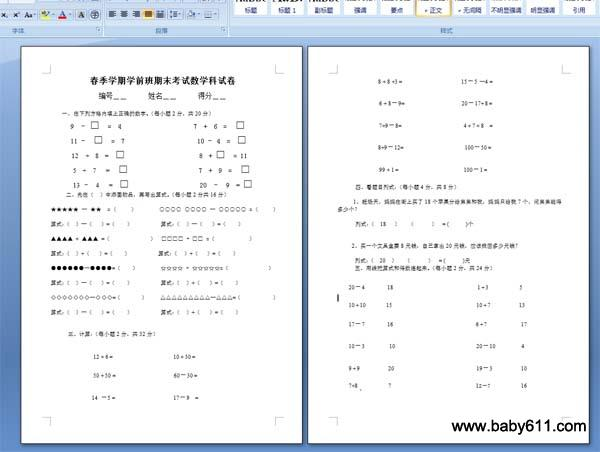春季学期学前班期末考试数学科试卷春季学期学前班期末考试数学科试卷编号＿＿      姓名＿＿      得分＿＿一、在下列方格内填上正确的数字。（每小题2分，共20分）9  － □  =  4                      7  +  6  =  □11 －  □  =  7                      10 － 4  =  □12  +  8  =  □                      8  + □  = 115  +  7   =  □                    7  +  9  =  □13 －  4   =  □                   20  －  9  = □二、先在（  ）中添画物品，再写出算式。（每小题2分共16分）★★★★★ - ★★  = （     ）         ○○○○ ○○○○ - ○○○○○ = （     ）算式：（  ）-（   ）=（    ）          算式：（  ）-（   ）=（    ）▲▲▲▲ + ▲▲▲ =（              ）  □□□□ + □□ =（            ）算式：（  ）+（   ）=（    ）          算式：（  ）+（   ）=（    ）●●●●●●-●●●●=（    ）        ☆☆☆☆ + ☆☆☆☆☆=（              ）算式：（  ）-（   ）=（    ）          算式：（  ）+（   ）=（    ）◇◇◇◇◇◇◇-◇◇◇=（       ）     △△△△△△△△△-△△△=（           ）算式：（  ）-（   ）=（    ）          算式：（  ）+（   ）=（    ）三、计算：（每小题2分，共32分）12 + 6 =       10 + 30 =50 + 50 =                60－30 =14 －5 =                17－9  =8 + 8 +3 =                   15－5 -4 =6 + 8－9=                   20－17+ 8 =7+9－8=                    4 + 7 + 8  =8+9－12=                      100－50 =99 + 1 =                  100－1 =四、看题目列式：（每小题4分，共8分）1、赶场天，妈妈在街上买了18个苹果分给弟弟和我，妈妈只给我7个，问弟弟能得多少个？

列式：（  18   ）   （      ）  = (      )个2、买一个文具盒要8元钱，自己拿出20元钱，应该找回多少元钱？

列式：（  20  ）   （      ）  = (      )元五、用线把算式和得数连起来。（每小题2分，共24分）20－4181 + 3510 + 101510 + 71317－7166 + 71710－31020－1049 + 9          2019－3         107+8           7                              12－7           168-

• 幼儿园试卷分类
• 将此试卷分享到：
• 数学最新更新